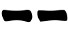## Tuesday, 17 May 2016

I had heard about the ancient game of Liubo but I didn't know it was related to the I Ching or to divination in general.

Sven Christensen sent me a very interesting article where a parallel between games and divination is drawn and some light on the relationship between Liubo and the I Ching is shed.

Liubo rules are long lost and they were most probably not directly related to divination with I Ching, so the best I can do is to make up a method for casting hexagrams using the same principles that Chris devised for his "One Die Method".

The picture below shows a Liubo dice wich is a 14-sided irregular polyhedron. Since the symbols are repeated twice, it is actually a 7 values die.

A possible method for casting a line (directly inspired to Chris' method) could be the following:
1. Throw the die and look at the face on the top:
2. If it contains lines, draw;
3. If it contains a symbol, draw;
4. If it is empty, throw the die again and look at the face on the top:
• If it contains lines, draw;
• If it contains a symbol, draw;
• If it is empty, restart from step 1
5. Repeat steps 1-4 until you have drawn all the lines from the bottom to the top of the hexagram.

#### Probabilites

Let's begin by noticing that the steps 1-4 above could not produce any line: the probability of such event and the probability of, instead, getting a line are:
Prob(no line) = 1/7 * 1/71/49
Prob(line) = 1- 1/4948/49

The probability for each possible outcome (conditioned to the event of actually getting a line) is:
Prob(6)  =   Prob(9) = (1/7 * 3/7) * 49/48  = 1/16 = 6.25%
Prob(8)  =  Prob(7) = 3/7 * 49/48             =  7/16 = 43.75%
Prob(yin) = Prob(yang) = 1/2

It is interesting to note that this methods has something in common with the Yarrow Stalk method (probabilities as multiple of 1/16, 49 possible outcomes from throwing) and something in common with three coins method (same probability for 9 and 6).

To enahance the fairness of the die, if you decide to build it, I suggest you alternate the symbols so that the the same symbol appears once on a square face and once on an hexagonal face.

3D printed Liubo dice are availble on Shapeways.

 http://www.livescience.com/52806-tomb-ancient-board-game-photos.html